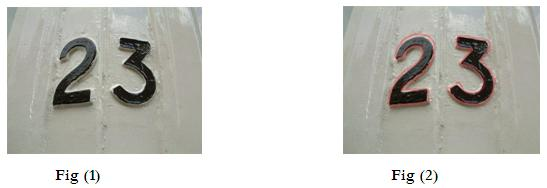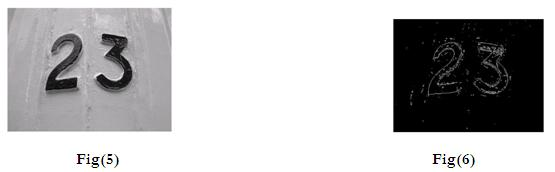# Currency Discrimination As an application of Image Processing – Neelam P.Kodrani

Title: Currency Discrimination As an application of Image Processing

Author: Neelam P.Kodrani, 4th Year B.E, Electronics and Communication Engineering Department

Abstract
This paper proposes the implementation of a very well known image processing algorithm, Image Segmentation, as currency discriminator. The idea is to capture the amount indicating character by a simple scan of currency and then recognition of the numerical data by edge detection and shape detection methods of image processing. The goal can be achieved by histogram matching of the detected image with the previously stored data. The examination of genuineness of the currency can also be tested with the help of optical sensors by examining security features of the currency. The approach is to bring advancement and reduce human workload.

Introduction
Daily growth in business leads to frequent deals with money. In order to perform quick operations of currency, the amount calculation must be fast efficient and accurate as well. Machination of currency detection, collection and discrimination leads to achieve the same. Use of Image Processing algorithms are one way of performing the task with high accuracy and satisfactory reliance. With the use of Image Segmentation and Edge detection algorithms the numbers printed on the currency can be read.

Operation
Firstly the authenticated data about currency is stored in the memory storage of a microcontroller device. Then currency to be calculated or discriminated is scanned through a digital camera. The image collected by that device is then processed under various algorithms of image processing. Using “Image Segmentation” the whole image of currency is divided into small segments. From that segments, then, using character recognition algorithm the segments which contains characters are filtered. After performing that we can obtain only that part of image that contains characters. Edge detection and histogram matching are used to detect which currency is scanned. The amount that the currency worth can be determined by comparing the preloaded data with obtained data.
Thus whole process is completed on the basis of image processing. With use of MATLAB, an IDE to implement image processing algorithms, the above described tasks can be performed.

Image segmentation
Image segmentation can be interpreted as the process of identification and filtration of the region of an image which contains logically predefined pixels.Let us assume that the image shown above in fig(1) needs to be segmented in two parts as shown in fig(2). The Numerical data indicated in the image needs to be abstracted from the image. The whole image to be segmented can be represented by R. Segmentation can be viewed as a process that partitions into R1, R2, R3….Rm. from which the region we require is say Rn.
There are many ways to achieve this. One of which is Edge Detection.

Edge Detection
Digital Image is made up of thousands of tiny parts which are called as pixels. A pixel is the smallest possible element of any image. All the pixels have their own gray value. At the very edge of any object in any image the pixel value changes very abruptly. This abrupt change in pixel value is detected with the help of gradients of images. Gradient is nothing else but derivative of image function with respect to x and y coordinates. Derivative in discrete domain is defined in terms of difference. Now where the pixel value is constant the derivative is zero. Where the pixel value changes uniformly the second derivative is zero. And for abrupt changes both the derivatives are nonzero. This fact is used in detecting the edges of objects in any images. This is done very easily using Hough Transform.

Hough TransformAs shown in fig(3) every point in a particular line can be expressed in th form of ρ(rho) and θ(theta) by equation given below.

ρ=xcosθ+ysinθ
Now if we represent every pixel in image by ρ and θ, then the points on that line will correspond to same values and no ρ and θ value but that will get maximum vote or will repeat itself maximum time. Thus we can obtain any particular line in an image. This Hough transform can be obtain in the MATLAb using following code.

bw = edge(r);
[H,T,R] = hough(bw);
figure(1), imshow(H,’XData’,T,’YData’,R,’fit’);
title(‘Hough Transform);Figure(6) is showing edges of the given objects in given image. Thus after obtaining this image by image subtraction technique, the character can be compared to preloaded data in the memory of microcontroller.

This whole embedded system can be developed and one currency discriminator can be built.

Application

Any place where cash transaction is done, this currency discriminator device can be put in order to operate rapidly as well as efficiently.

REFERENCES

R. C. Gonzalez and R. E. Woods, 2009, Digital.Image.Processing, 3rd Edition, Pearson, Delhi, India, 976p.
www.coursera.org/course/images
www.wikipedia.org/wiki/Currency_detector
www.wikipedia.org/wiki/Indian_rupee